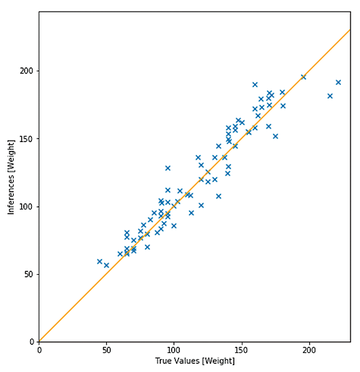# On the Edge

#### On the Edge

The previous section introduced the basics for solving a regression problem applied to lettuce weight prediction and then showed how to convert a model to the TensorFlow Lite format after training and then optimize. Now I am ready to deploy the trained optimized model (`model.tflite`) to the brain (i.e., the Raspberry Pi) of the vertical farm.

Remember that on IoT devices, only the standalone and lightweight TensorFlow Lite Runtime needs to be installed. As on the R&D computer, I used Python 3.7 and `pip` to install the TensorFlow Lite Runtime wheel package. In this case, the Raspberry Pi is running Raspbian Buster, so I install the Python wheel as follows:

`pip3 install https://dl.google.com/coral/python/tflite_runtime-2.1.0-cp37-cp37m-linux_armv7l.whl`

Then, executing the model and making a prediction with the Python API is quite easy. Because I am working with real-world data from sensors, it is crucial to implement strong preprocessing techniques to sample, filter, and normalize the data fed into the neural network.

The first time through, I need to allocate memory for the tensors:

```import tflite_runtime.interpreter as tflite
interpreter = tflite.Interpreter(model_content=tflite_model_ffile)
interpreter.allocate_tensors()```

Next, I feed the input tensors with the input features, invoke the interpreter, and read the prediction. The code snippet in Listing 4 has array `input_tensor`, which contains the input features (i.e., the preprocessed CumLight, CumTemp, etc.), and `tensor_index`, which corresponds to the number of features.

Listing 4

Weight Prediction

```interpreter.set_tensor(tensor_index=9, value=input_tensor)
# run inference
interpreter.invoke()
# tensor_index is 0 because the output contains only a single value
weight_inferred = interpreter.get_tensor(tensor_index=0)```

#### Results

The time has come to evaluate the accuracy of the model and see how well it generalizes with the test set, which I did not use when training the model. The results will tell me how good I can expect the model prediction to be when I use it in the real world.

First, I can evaluate the accuracy at a glance with a graph (Figure 5). The blue crosses show the inferred weight values as a function of the true values for the test dataset. The error can be seen as the distance between the blue crosses and the orange line.Figure 5: The model error can be found with a test dataset.

A metric often used to evaluate regression models is the mean absolute percentage error (MAPE), which measures how far predicted values are from observed values. For the test dataset, the MAPE=9.44%, which is definitely precise enough for a vertical farmer.

#### Execution Time

For evaluation purposes, I installed the full TensorFlow distribution on a test Raspberry Pi and compared the inference execution time between the original TensorFlow model and the optimized `.tflite` model with the TensorFlow Lite Runtime. The results showed that inference with TensorFlow Lite ran in the range of a few milliseconds and was three to four times faster than TensorFlow.

Express-Checkout as PDF
Price \$2.95
(incl. VAT)